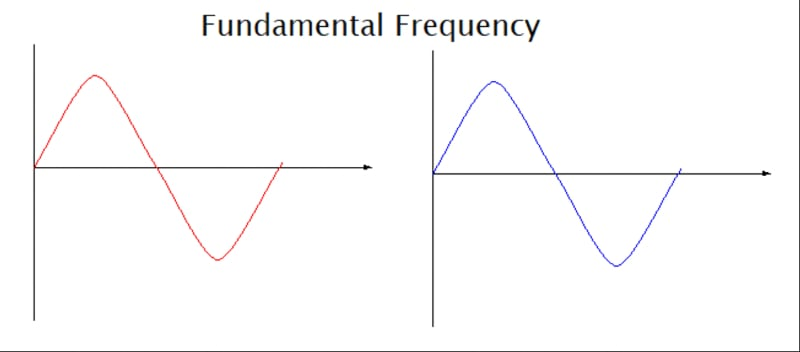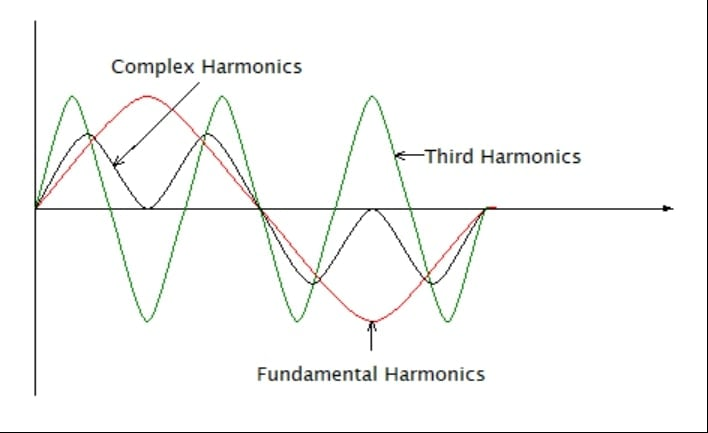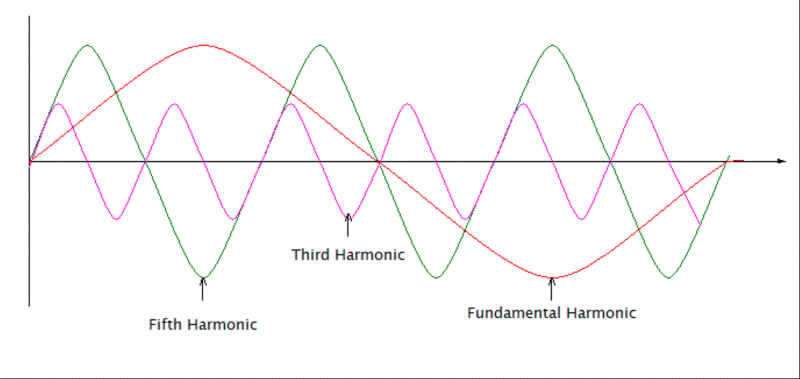Technical Article

# Mitigating Harmonics in Power Systems

May 04, 2023 by Simon Mugo

## This article will guide engineers in understanding harmonics, causes, types, equations, and sequences and mitigate harmonics effects.

Harmonics are fundamental frequency multiples that have existed since the beginning of the 20th Century when engineers and scientists discovered discontinuous loads, which came as a result of the vacuum tube invention.

In the beginning, harmonics effects were negligible, and most engineers ignored them. As technology grew and with the invention of sophisticated electronics like electronic lighting, uninterruptible power supplies, programmable logic controllers, and variable frequency drives, harmonics injected power quality challenges. The effects of harmonics on the quality of signals produced by this equipment triggered changes in designs, filtering processes, and installation procedures.

Despite engineering changes and general awareness, harmonics still need to improve. This article will empower you with key knowledge to mitigate harmonics.

### Defining Harmonics

In an electrical power system, harmonics can be defined as the multiple of the current or voltage at the fundamental voltage frequency. Anytime you observe a waveform, and it deviates from the expected sinewave shape, it contains harmonics.

### Causes of Harmonics

Linear or nonlinear AC signals are categorized according to how the systems draw power from the supply source. Harmonics are caused by the nonlinear systems which draw currents in short, abrupt pulses. The drawn pulses disrupt the waveforms of the current by causing distortion. The distortion generates harmonics which lead to power problems, affecting the load and the distribution system. Examples of nonlinear load systems include electronic devices like TVs.

### Fundamental Electrical Harmonics

This is where power originates from the generator. Its frequency is referred to as fundamental frequency or first harmonic frequency. Its value is either 50 Hz or 60 Hz, depending on your country’s choice. All electrical and electronic systems are made to work well under this frequency.### Harmonics Orders and Complex Waveforms

#### Second-order Harmonics

Second-order harmonics are waveforms with frequencies at 100 Hz – that is, 50 Hz multiplied by two. This is an indication that the second harmonics have a frequency twice the fundamental frequency. Below are the waveforms for the second harmonics.##### Figure 2. Second-order harmonics waveform demonstration. Image used courtesy of Simon Mugo

From the waveform graph above, when the fundamental harmonics get to zero, it gets to its high value, and so on. This is the reason the second harmonic initiates the reverse direction, implying the negative sequence current flows in the given electrical circuit. The negative sequence current affects the induction motor, where it opposes the rotating magnetic field. The result of the opposition is that the motor produces lower mechanical torque than expected. This type of harmonic is also known as the negative sequence.

#### Third-order Harmonics

This has a frequency triple that of the fundamental harmonic. The frequency is 150 Hz. This is a very dangerous type of harmonic. Below is its waveform.##### Figure 3. Third-order harmonics waveform. Image used courtesy of Simon Mugo

From the graph, both third and fundamental harmonics currents reach zero at the same time. They both get high at the same value, but the points are opposite each other. This action makes the harmonics create a zero-sequence current, leading to an increase in the power system’s neutral voltages. Increasing the neutral voltage causes the relay to operate a circuit breaker. This effect is caused by the third harmonic current. The third harmonic is also known as triplens.

#### Fourth-order Harmonics

This has a frequency of 200 Hz, which is four times the fundamental frequency. Below is the figure of the waveforms.##### Figure 4. Fourth harmonic waveforms. Image used courtesy of Simon Mugo

When the fundamental harmonic current gets to the highest value, the fourth harmonic does the same too. From the graph, this is true for both the negative and the positive sides. This is why such harmonics increase the current that flows in a conductor, which increases the equipment temperature. It is also known as positive harmonic.

#### Fifth-order Harmonics

Fifth-order harmonics have a frequency of 250 Hz and characteristics similar to third-order harmonics but with a higher operating frequency. Below are the waveforms for the harmonic.### Waveform Analysis

From the waveforms above, it is clear that a complex waveform comprises a combination of the harmonics and fundamental waveform, each having its phase angles and pick values.

For a simple example, if we have the fundamental frequency given as E=Vmax(2πft) we can calculate the values of the harmonics as shown below.

#### Second Harmonics

$E_{2}=v_{2(max)}(2\times2\pi ft)$

$E_{2}=v_{2(max)}(4\pi ft)$

$E_{2}=v_{2(max)}(2\omega t)$

#### Third Harmonics

$E_{3}=v_{3(max)}(3\times3\pi ft)$

$E_{3}=v_{3(max)}(6\pi ft)$

$E_{3}=v_{3(max)}(3\omega t)$

#### Fourth Harmonics

$E_{4}=v_{4(max)}(4\times2\pi ft)$

$E_{4}=v_{4(max)}(8\pi ft)$

$E_{4}=v_{4(max)}(4\omega t)$

Where 2πf=ω

This process goes on and on for higher orders of harmonics.

Therefore, the equation for the complex waveform can be deduced as

$E_{T}=E_{1}+E_{2}+E_{3}+............+E_{n}$

### Harmonic Sequencing

Below is a summary of the harmonic sequencing, demonstrating how the frequency changes from fundamental frequency to higher orders.

##### Table 1. Table of Harmonic Sequence
 DESIGNATION Fund. 2nd 3rd 4th 5th 6th 7th 8th 9th Frequency in Hz 50 100 150 200 250 300 350 400 450 Sequence + - 0 + - 0 + - 0

Some systems use the 60 Hz fundamental frequency. The same harmonics apply under a similar calculation method.

##### Table 2. Harmonic Effects
 SEQUENCE ROTATION EFFECT OF HARMONIC + Forward Overheating affects - Reverse Reduction of the motor torque 0 None Causes heating by adding voltage and currents in the neutral wires.

### Harmonics Summary

From the article, it is clear that:

• Harmonics is the deviation of the fundamental frequency in multiples of two or more.
• Harmonics leads to an increase in heat generated by a system, the amount of voltage currently released by an object, and it affects the torques released by motors.
• Fundamental harmonics have a frequency of 50 Hz or 60 Hz, depending on the country’s choice.
• Harmonics is defined as the multiple of the current or voltage at the fundamental frequency.
• Fundamental electrical harmonics is where power originates from the generator and its frequency. The frequency at fundamental electrical harmonics is referred to as fundamental frequency.
• Second-order harmonics have frequencies of 100 Hz – or 50 Hz, the value of fundamental frequency multiplied by two.
• Third-order frequency is triple the fundamental frequency meaning its frequency is 150 Hz.

Featured image used courtesy of Adobe Stock